Change List for this Rule

General Order 95

Appendix F

Typical Problems

Part III

 To maintain poles in proper position at angles and corners, it is generally necessary to use guys or some other form of pole bracing. Unless the line is deadended, the pull of the conductors is taken as being the same throughout the line. The degree of unbalanced pull at an angle or corner pole is dependent upon the angle in the line at that point; that is, the greater the angle in the line, the greater is the magnitude of unbalance. Rule 47 specifies that when the longitudinal loads in a structure are not normally balanced, the members stressed shall be of such strength as to withstand the total unbalanced load with factors of safety equal to those of Table 4. As it is assumed that the line considered in this problem is Grade A construction, the pole would be required to provide a safety factor of 4 against unbalanced loads; where guys are used to take the unbalanced loads they must provide a safety factor of 2. It is assumed that the line discussed in the foregoing deadend problem crosses from one side of a street to the opposite side, that the longitudinal distance along the street between the two poles concerned is 77 feet, and that the angle of deviation is 33° (see sketch). This would result in an unbalanced force being exerted in the direction of A of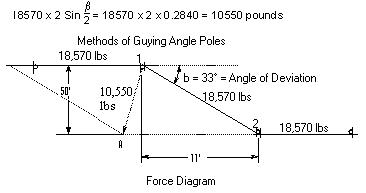Assuming the pole height and framing as shown inPart 2 , the top circumference of pole to be 25, the ground circumference to be 50 and the center of load to be 37.4 feet above ground line (as determined in Part 2 ), the fiber stress on the pole at the ground line is as follows: Bending moment, M = 37.4 x 10,550 = 394,400 poundfeet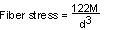where circumference = 50 and d = 15.9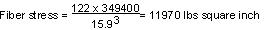As a safety factor of 4 is required, the allowable working stress is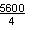or 1,400 lbs per square inch, and therefore the use of guys is necessary. A single guy attached at the center of load could be used provided the modulus of rupture with a safety factor of 4 is not exceeded. The stress due to guying at this point is as follows: Bending moments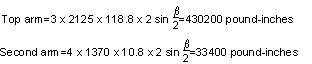Total moment=463600 pound-inches Section modulus, E The section modulus (E) at 37.4 feet above ground is 89.6 inches cubed, which is the value computed in Problem 2 Fiber stress: The fiber stress is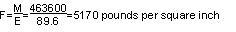Since this stress exceeds the allowable stress of 1,400 lbs per sq. in. for the pole, it is necessary to place guys at more than one point on the pole, and therefore, they are attached at positions similar to the guys shown in the diagram on Page F22.

Note:      Revised February 5, 2014 by Decision No. 14-02-015.